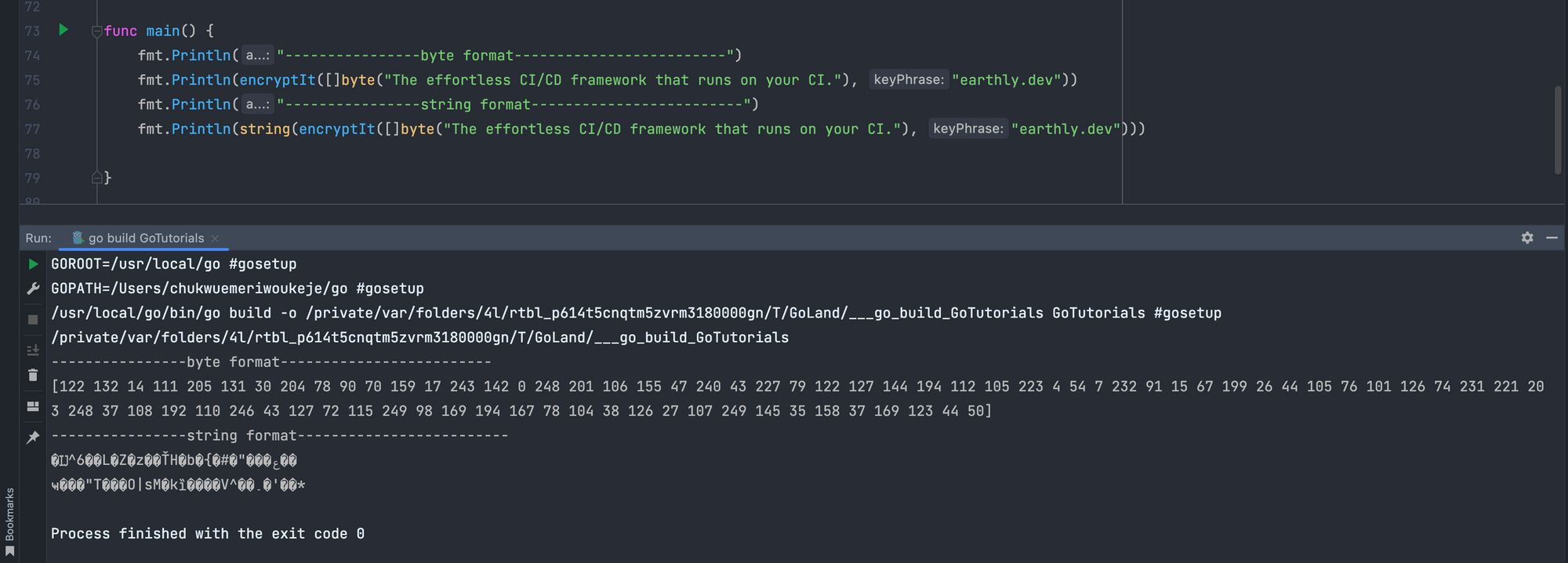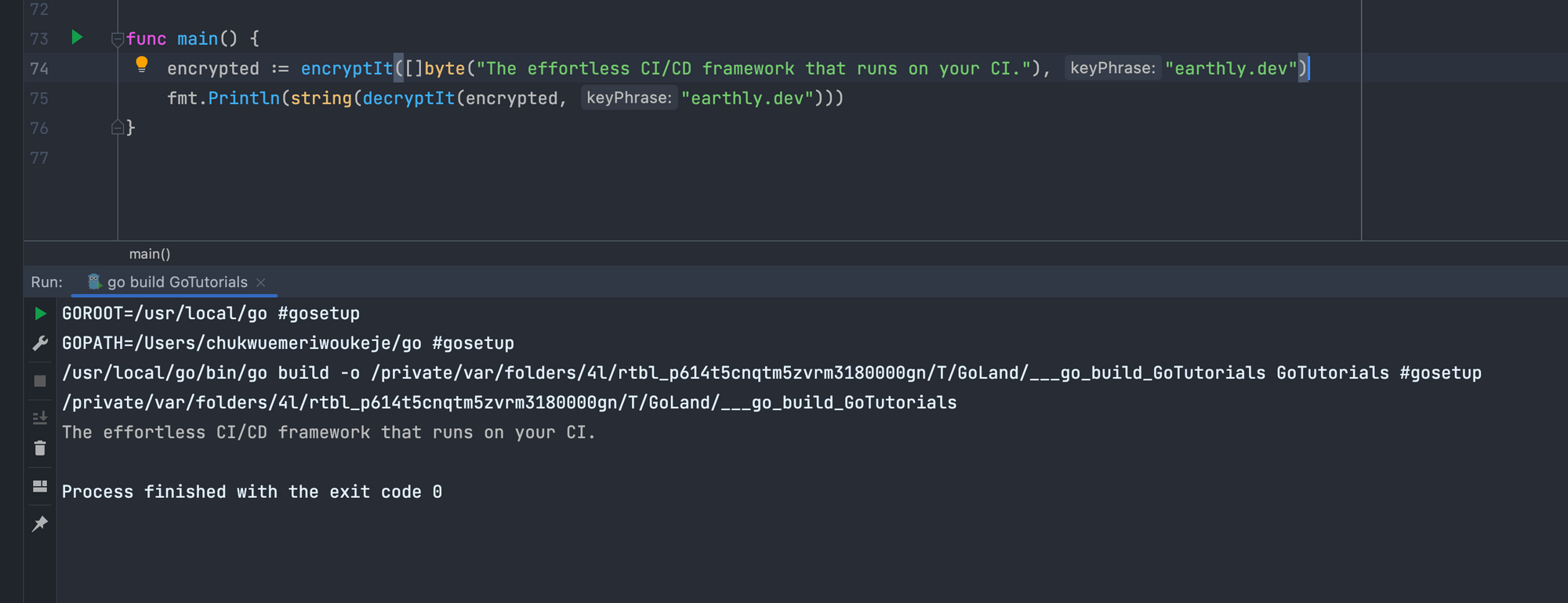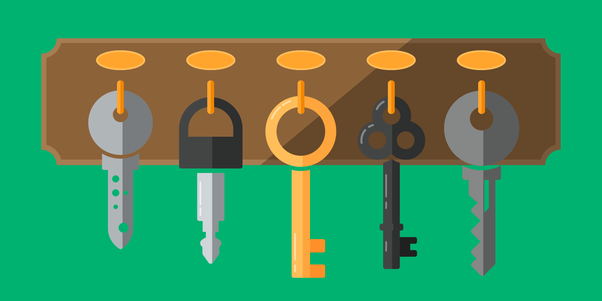# Cryptography & Encryption in Go

Ukeje Goodness

The article summarizes Go cryptography techniques. Earthly streamlines and speeds up Go build pipelines. Check it out.

One of the biggest concerns for modern web developers is security. Whether your goal is protecting a user’s personal data, effectively authenticating a user’s identity, or securing company databases, cryptography, and encryption can help.

Cryptography is the study of techniques for secure communication between a sender and an intended recipient. Cryptographic techniques employ mathematical functions to secure data using various algorithms and systems.

The Go programming language provides a `crypto` package for cryptography-related operations in the standard library. You can use the Crypto package for many functionalities like encryption, hashing, cryptographically generated random numbers, and much more.

This tutorial will help you understand cryptography concepts and how to implement them in the Go programming language.

## Hashing Functions in GoHashing is a data protection technique for transforming given inputs into another value (the hash value) of fixed length using a hashing algorithm. Hashing algorithms use mathematical functions to transform any length of data into a fixed-length.

Good hashing algorithms are one-way functions such that the original input cannot be retrieved. To validate data with a hashing algorithm , the input is hashed and compared to the hashed value. When you’re building an application, you’ll have to store the hashed value in a datastore, and for validation, you’ll hash the user’s input and compare the two hashes.

There are many hashing algorithms in use; the most popular ones are the SHA (Secure Hash Algorithm) and the MD(Message Digest) Algorithms.

### Hashing in Go using the MD5 Algorithm

The MD5 algorithm is one of the successors of the MD2 algorithm. The MD2 and MD5 algorithms have similar architectures except that the MD5 algorithm was build specifically for 32bit systems, and the MD2 algorithm was built for 8-bit systems.

While the structures of these algorithms are somewhat similar, the design of MD2 is quite different from that of MD4 and MD5. MD2 was optimized for 8-bit machines, whereas MD4 and MD5 were aimed at 32-bit machines. The Message Digest Method 5 Algorithm (MD5) is a cryptographic algorithm that returns a 128-bit digest from any text represented as 32-digit hexadecimal.

We’ll start by importing two packages. The md5 algorithm package, which is a subpackage of the `crypto` package, and the hex package, which we will use for encoding the hexadecimal returned from the MD5 algorithm to a string.

``````import (
"crypto/md5"
"encoding/hex"
)``````

Next we can create an `mdHashing` function that takes in an input and returns the encoded string of the hash. The input is converted to a slice of bytes using the built-in `byte` function and then hashed using the `Sum` method of the `md5` package.

``````func mdHashing(input string) string {
byteInput := []byte(input)
md5Hash := md5.Sum(byteInput)
return hex.EncodeToString(md5Hash[:]) // by referring to it as a string
}``````

Using the `EncodeToString` function, you can return a string format of the hash by passing in the hashed byte slice.### Hashing in Go using the SHA256 Algorithm

The SHA256 (Secure Hash Algorithm 256-bits) is a cryptographic one-way hashing algorithm that returns a 256-bit hash value. Like the MD5 algorithm, the SHA256 algorithm is a key-less hashing function.

You’ll need to import the `sha256` package from the `crypto` package for use. And just like the MD5 algorithm example, you’ll also need to import the `hex` package to encode the hash value to a string.

``````import (
"crypto/sha256"
"encoding/hex"
)``````

Then we can create a `ShaHashing` function that takes in a string as input and returns a string of the hash value. Just like before, the input is converted to a slice of bytes, and then, using the `Sum256` method of the `sha256` package, the `plainText` variable is hashed, and a string hash value is returned.

``````func shaHashing(input string) string {
plainText := []byte(input)
sha256Hash := sha256.Sum256(plainText)
return hex.EncodeToString(sha256Hash[:])
}``````Hashing Algorithms are usually one-way functions; thus, you can’t use them for communication since you can’t retrieve the original value; you’ll be comparing the hash value you stored to the value of input most of the time. For communication-related purposes, you can use encryption algorithms to relay messages securely.

## What Are Encryption and DecryptionEncryption is a data protection technique of encoding(ciphering) data with the intent of decoding(deciphering) it for later use.

Ciphers are the result of using an encryption algorithm on a plain literal. When you use a cryptographic function on plain text, you’ll need the same algorithm, and encryption keys to decipher the cipher text.r. Encryption is popularly used for files and messages since the initial state of the data is still essential.

Decryption is the reverse process of encryption where you use the same cryptographic key and encryption algorithm to return the cipher text to the original state.

## Encrypting Text in Go

There are many encryption algorithms available for you to use; the most popular and one of the strongest is the AES algorithm. The AES(Advanced Encryption Standard) algorithm uses a key length of 128 bits for encryption and decryption and returns a 128-bit cipher.

You can encrypt data in Go using the `aes` and cipher packages.

``````import (
"crypto/aes"
"crypto/cipher"
)``````

You will also need a key phrase for the cipher. The `aes` package will use the key phrase and a nonce to encrypt the data, and you’ll need the key phrase and nonce to decrypt the data. In this tutorial, you will use the md5 hash function to generate a key phrase for the encryption.

Start by creating a function called `encryptIt`

``````func encryptIt(value []byte, keyPhrase string) []byte {

}``````

The `encryptIt` function takes in a byte slice and a string key phrase and returns a byte slice.

``````func encryptIt(value []byte, keyPhrase string) []byte {

aesBlock, err := aes.NewCipher([]byte(mdHashing(keyPhrase)))
if err != nil {
fmt.Println(err)
}
}``````

First, you create an AES block using the `NewCipher` method. The `NewCipher` method takes in the keyphrase, which, in this case, you hashed for increased security.

The next step is to create a new cipher with a nonce. You can use the Galois Counter Mode(GCM) using the `NewGCM` method that takes in the AES block.

``````func encryptIt(value []byte, keyPhrase string) []byte {

aesBlock, err := aes.NewCipher([]byte(mdHashing(keyPhrase)))
if err != nil {
fmt.Println(err)
}

gcmInstance, err := cipher.NewGCM(aesBlock)
if err != nil {
fmt.Println(err)
}
}``````

The `NewGCM` method returns a 128-bit block cipher with a nonce length.

You can now create a nonce of the length specified in the `NewGCM` method.

`````` nonce := make([]byte, gcmInstance.NonceSize())

The `nonce` variable you declared is a byte slice of the nonce size from the `NewGCM` method. You can now read the byte length into the nonce using the `ReadFull` method of the `io` package, where an instance of a cryptographically generated random number will be passed to the nonce.

The final step is to encrypt the plain-text using the nonce. The `Seal` method of your Galois counter mode instance encrypts the plain text and returns a slice of bytes.

`````` cipheredText := gcmInstance.Seal(nonce, nonce, value, nil)

return cipheredText``````

You could pass in additional data to the `Seal` method instead of `nil` as seen above. The function returns the byte slice ciphered text from the `Seal` method.## Decrypting Ciphered Text in Go

You’ll have to use the same encryption algorithm, keyphrase, and nonce to decrypt the cipher. The `decryptIt` function takes in the ciphered text `cipheredText` and the keyphrase and returns a slice of byte that we can convert to a readable string format for use.

``````func decryptIt(ciphered []byte, keyPhrase string) []byte {

}``````

You must use a function because it might be expensive to store the encrypted text. In this case, the `decryptIt` function will take in the output from the `encryptIt` function.

``````  hashedPhrase := mdHashing(keyPhrase)
aesBlock, err := aes.NewCipher([]byte(hashedPhrase))
if err != nil {
log.Fatalln(err)
}
gcmInstance, err := cipher.NewGCM(aesBlock)
if err != nil {
log.Fatalln(err)
}``````

You’ve may have noticed that the code above is the same as in the `encryptIt` function; you hashed the keyphrase for decryption, created an AES block, and a new cipher instance.

You now need to remember the nonce you used for encryption, and you can get that using the `NonceSize` method. You can get the cipher text you need to decrypt by slicing the nonce off the cipher slice

``````nonceSize := gcmInstance.NonceSize()
nonce, cipheredText := ciphered[:nonceSize], ciphered[nonceSize:]``````

Now that you have the cipher without the nonce, you can use the `Open` method of your GCM instance to decrypt the cipher. The open method takes in the nonce, cipher, and additional parameters.

`````` originalText, err := gcmInstance.Open(nil, nonce, cipheredText, nil)
if err != nil {
log.Fatalln(err)
}
return originalText``````

The function returns the original text from the `Open` method, as you can see below.## Generating Cryptographically Secure Random Values in GoThere’s a high probability that when you’re building an app, you want to generate random values for different purposes. Generating random values using the `random` package isn’t as random as it may seem. The Authors of Go knew this and provided a `rand` package in the `crypto` package for generating cryptographically secure random values.

Here’s how you can generate cryptographically secure random numbers in Go. Start by creating aThe `generateCryptoRandom` function that takes in a string from where the random string it returns will be generated and a 32—bit integer for the length of the random string you want to generate.

``````func generateCryptoRandom(chars string, length int32) string {

}``````
`````` bytes := make([]byte, length)

The `bytes` variable is a new byte slice of the length of the random values you want as output. The `Read` method of the `rand` package reads random bytes into the `byte` slice.

``````for index, element := range bytes {
randomize := element%byte(len(chars))
bytes[index] = chars[randomize]
}

return string(bytes)``````

The range `forloop` loops through the `bytes` slice and sets the values of the index in the byte slice equal to a random position `randomize` by getting the remainder when the element of the byte slice divides the length of the characters you’re generating a random value from.

The cryptographically secure random values are in a byte slice, and you can use the `string` function to convert it to a string for the function.

## Conclusion

In this tutorial, we looked at increasing application security using cryptographic techniques. Using encryption, hashing algorithms, and secure random value generation, we’ve delved into how to construct more secure Go applications, even incorporating JWT for authentication.

As you continue to build your secure Go app, you might also want to consider streamlining your build process. For that, you can give Earthly a go. It’s an excellent tool for simplifying and optimizing your build automation.

Earthly Cloud: Consistent, Fast Builds, Any CI
Consistent, repeatable builds across all environments. Advanced caching for faster builds. Easy integration with any CI. 6,000 build minutes per month included.

Ukeje Goodness

Goodness is a technical writer and backend developer(Go) simplifying various technology topics as he explores this fascinating field.

Updated:

Published: